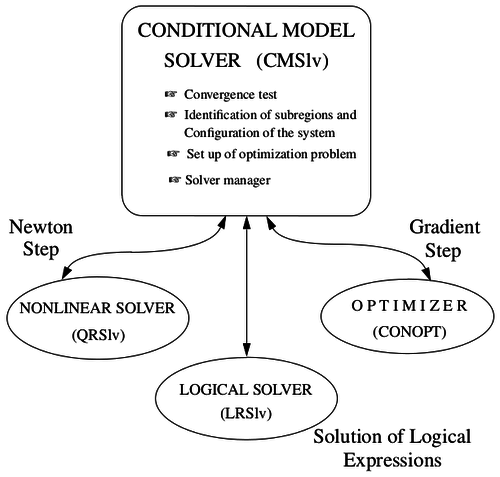# CMSlv

NLA
QRSlv
CMSlv
IPSlv
NLP
CONOPT
IPOPT
TRON
MINOS
Opt
NGSlv
DAE/ODE
IDA
LSODE
DOPRI5
LA
Linsolqr
Linsol
LP
MakeMPS
Logic
LRSlv

This is the conditional modelling solver implemented by Vicente Rico-Ramirez. Currently this solver is fully operational -- it solves the sample models given in Vicente's thesis, and these have been incorporated in the the TestSuite. You need to have CONOPT installed before CMSlv will work; it's used as part of the boundary-crossing algorithm. CMSlv also makes use of a subsidiary logical-expression-solvers called LRSlv.Implementation of CMSlv (from Vicente Rico-Ramirez' thesis, see our publications).

## Example

Here is an extract of a full example called models/linmassbal.a4c:

```(* Boundaries *)
CONDITIONAL
cond1: Fmain <= B;
cond2: Fmain >= B;
END CONDITIONAL;

bol1 == SATISFIED(cond1,1e-08{lb_mole/hour});
bol2 == SATISFIED(cond2,1e-08{lb_mole/hour});

(* Variant Equations *)
eq1a: Fsub1 = a * Fmain;
eq1b: Fsub2 = b * Fmain;
eq2a: Fsub1 = a * Fmain;
eq2b: Fsub2 = b * Fmain;
eq3a: Fsub1 = a * Fmain;
eq3b: Fsub2 = b * Fmain;

(* Disjunctive Statements *)
WHEN (bol1,bol2)
CASE TRUE,FALSE:
USE eq1a;
USE eq1b;
CASE FALSE,FALSE:
USE eq2a;
USE eq2b;
CASE FALSE,TRUE:
USE eq3a;
USE eq3b;
END WHEN;
```